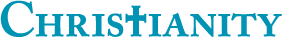## What percentage of my answers are accepted?

0

(click on this box to dismiss)

Returns the total number of answers, the number of accepted answers, and what percentage of answers are accepted.Q&A for committed Christians, experts in Christianity and those interested in learning more

``````-- What percentage of my answers are accepted?
-- Returns the total number of answers, the number of accepted answers, and what percentage of answers are accepted.

SELECT
Sum(CASE WHEN q.AcceptedAnswerId IS NULL THEN 0 ELSE 1 END) AS [Accepted Answers],
Round(Sum(CASE WHEN q.AcceptedAnswerId IS NULL THEN 0 ELSE 1 END) * 100.0 / Count(a.Id), 1) AS [Percentage Accepted]
FROM
Posts AS a
LEFT JOIN
Posts AS qHold tight while we fetch your results